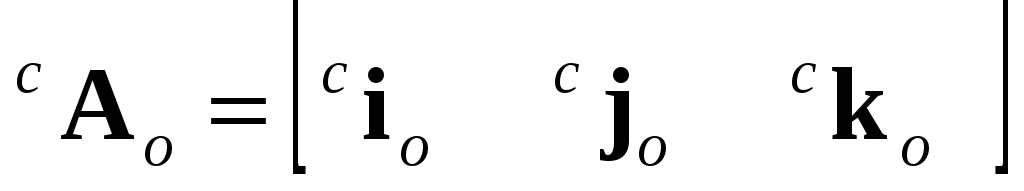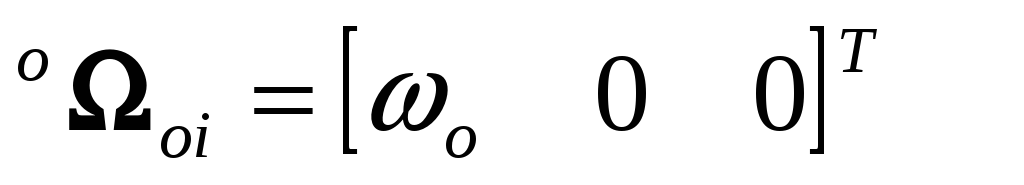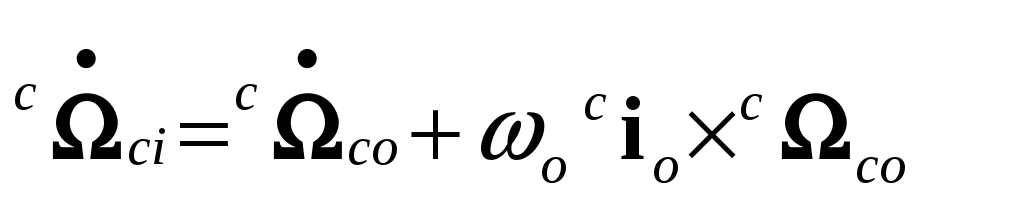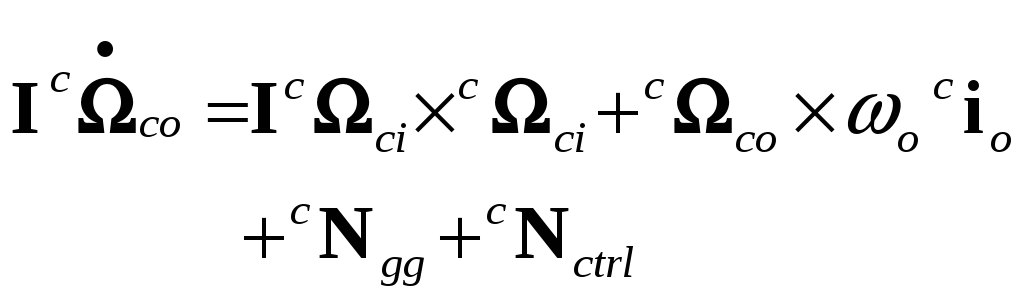# Pedro Tavares, Paulo Tabuada, ‡

 whereis the direct cosine matrix which transforms vectors expressed in the Orbit CS to the Control CS. Small satellites are usually launched into polar orbits with small eccentricities. Therefore, the angular velocity of the Orbital CS w.r.t. the Inertial CS is approximately given by:(7) The derivative of eq. (6) now becomes:(8) substituting in the dynamics equation (1) and neglecting the disturbance torque we get:(9) Download 1.52 Mb.Share with your friends: